1. Home
2. Docs
3. Numerical Methods
4. Interpolation and Regression
5. Lagrange Interpolation

# Lagrange Interpolation

## Introduction

We can write the second order polynomial in the form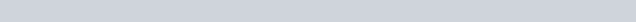Let us assume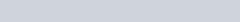are the three interpolating points then,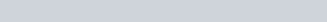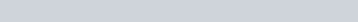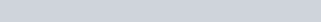Using above we have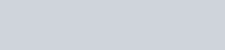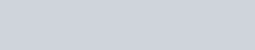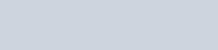Putting the values together we have,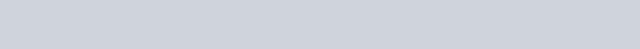This form can be written aswhere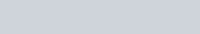Generalizing we have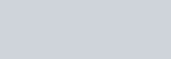## Algorithm

1. Start
2. Read number of points, let us assume (n)
3. Read the value at which the value is needed, let us assume (x)
5. Calculate the value of Li
for I = 1 to n
for j = 1 to n
if (j!=i)
L[i] = L[i]*((x-x[i])/(x[i]-x[j]))
Endif
endfor
endfor
6. Calculate the interpolated point at x
For i= 1 to n
t=t+fx[i]*lx[i]
Endfor
7. Print the interpolated value v at x
8. End


## Codes

C ProgramJS
#include <stdio.h>
#include <conio.h>

void main()
{
float x, y, xp, yp = 0, p;
int i, j, n;
clrscr();
/* Input Section */
printf("Enter number of data: ");
scanf("%d", &n);
printf("Enter data:\n");
for (i = 1; i <= n; i++)
{
printf("x[%d] = ", i);
scanf("%f", &x[i]);
printf("y[%d] = ", i);
scanf("%f", &y[i]);
}
printf("Enter interpolation point: ");
scanf("%f", &xp);
/* Implementing Lagrange Interpolation */
for (i = 1; i <= n; i++)
{
p = 1;
for (j = 1; j <= n; j++)
{
if (i != j)
{
p = p * (xp - x[j]) / (x[i] - x[j]);
}
}
yp = yp + p * y[i];
}
printf("Interpolated value at %.3f is %.3f.", xp, yp);
getch();
}